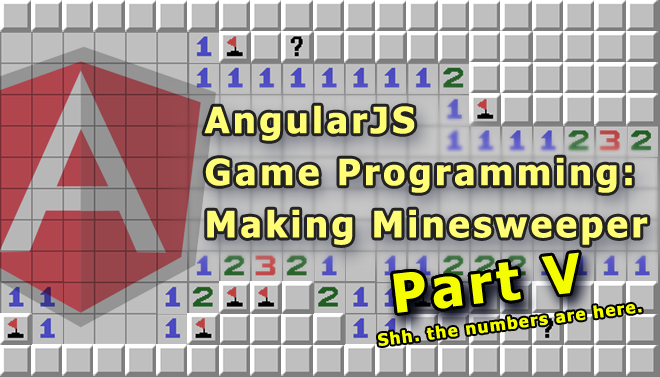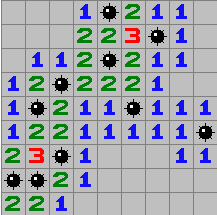# AngularJS Game Programming: Making Minesweeper (Part V)Last time on “AngularJS Game Programming”: We added mines! In this post we’re going to add numbers, doesn’t seem quite as cool as things that boom but it does get us that much closer to having our own complete version of minesweeper.

#### Numbers

So far so good. Now we let’s add the numbers. We do this by going through each spot, checking all the spots around it and counting the mines. Let’s start by writing the function to calculate the number for a single spot.

Only spots that are currently empty that have mines around them should be populate with a number, otherwise they should be left as “empty”. Spots that have a mine should be skipped.

When we count the number of mines around a spot we should be careful about going off the grid. For example if we’re checking the spot at the very top left, there are no spots to the left or above it. If we try to access it we will get an error.

Taking all that into account our function should look like this:

```function calculateNumber(minefield, row, column) {
var thisSpot = getSpot(minefield, row, column);

// if this spot contains a mine then we can't place a number here
if(thisSpot.content == "mine") {
return;
}

var mineCount = 0;

// check row above if this is not the first row
if(row > 0) {
// check column to the left if this is not the first column
if(column > 0) {
// get the spot above and to the left
var spot = getSpot(minefield, row - 1, column - 1);
if(spot.content == "mine") {
mineCount++;
}
}

// get the spot right above
var spot = getSpot(minefield, row - 1, column);
if(spot.content == "mine") {
mineCount++;
}

// check column to the right if this is not the last column
if(column < 8) {
// get the spot above and to the right
var spot = getSpot(minefield, row - 1, column + 1);
if(spot.content == "mine") {
mineCount++;
}
}
}

// check column to the left if this is not the first column
if(column > 0) {
// get the spot to the left
var spot = getSpot(minefield, row, column - 1);
if(spot.content == "mine") {
mineCount++;
}
}

// check column to the right if this is not the last column
if(column < 8) {
// get the spot to the right
var spot = getSpot(minefield, row, column + 1);
if(spot.content == "mine") {
mineCount++;
}
}

// check row below if this is not the last row
if(row < 8) {
// check column to the left if this is not the first column
if(column > 0) {
// get the spot below and to the left
var spot = getSpot(minefield, row + 1, column - 1);
if(spot.content == "mine") {
mineCount++;
}
}

// get the spot right below
var spot = getSpot(minefield, row + 1, column);
if(spot.content == "mine") {
mineCount++;
}

// check column to the right if this is not the last column
if(column < 8) {
// get the spot below and to the right
var spot = getSpot(minefield, row + 1, column + 1);
if(spot.content == "mine") {
mineCount++;
}
}
}

if(mineCount > 0) {
thisSpot.content = mineCount;
}
}

```

Now that we have the function we need to call it for every spot on the map, which we can do with this function:

```function calculateAllNumbers(minefield) {
for(var y = 0; y < 9; y++) {
for(var x = 0; x < 9; x++) {
calculateNumber(minefield, x, y);
}
}
}

```

Now we update `createMinefield()` to call `calculateAllNumbers()`, and that only leaves the display. The display is very similar to the code we already have, we just need to add one for every number (1 through 8).

```<td ng-repeat="spot in row.spots" ng-click="spot.isRevealed = true">
<img ng-if="!spot.isRevealed" src="block.png">
<img ng-if="spot.isRevealed && spot.content == 'empty'" src="empty.png">
<img ng-if="spot.isRevealed && spot.content == 'mine'" src="mine.png">
<img ng-if="spot.isRevealed && spot.content == 1" src="number-1.png">
<img ng-if="spot.isRevealed && spot.content == 2" src="number-2.png">
<img ng-if="spot.isRevealed && spot.content == 3" src="number-3.png">
<img ng-if="spot.isRevealed && spot.content == 4" src="number-4.png">
<img ng-if="spot.isRevealed && spot.content == 5" src="number-5.png">
<img ng-if="spot.isRevealed && spot.content == 6" src="number-6.png">
<img ng-if="spot.isRevealed && spot.content == 7" src="number-7.png">
<img ng-if="spot.isRevealed && spot.content == 8" src="number-8.png">
</td>

```

If you click on each block you should get something like this:Play with it here: http://jsfiddle.net/luisperezphd/m3R3W/

### Next Time

In the next post we’re going to add logic to detect whether you’ve won.

Next Post: Detecting if the player won.

1.says
•Luis Perez says